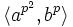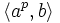# Sylow subgroup of holomorph of cyclic group of odd prime-cube order

## Definition

Let$p$ be an odd prime number. This group is defined as the$p$-Sylow subgroup of the holomorph of the cyclic group of order$p^3$. Equivalently, it is the semidirect product of the cyclic group of order$p^3$ and the cyclic group of order$p^2$, where the generator of the latter acts on the former by the$(p+1)$-power map.

It can be given by the explicit presentation:$G := \langle a,b \mid a^{p^3} = b^{p^2} = e, bab^{-1} = a^{p + 1}\rangle$

Note that the case$p = 2$ is different, because the automorphism structure of the cyclic group of order$2^3$ is different, with a non-cyclic 2-Sylow subgroup of automorphisms. See holomorph of Z8 for details on that group.

## Arithmetic functions

Function Value Explanation
order$p^5$ semidirect product of groups of order$p^3$ and$p^2$
exponent$p^3$ element$a$ has order$p^3$, no element of order$p^4$
derived length 2 commutator subgroup$\langle a^p \rangle$ cyclic of order$p^2$
nilpotency class 3
Frattini length 3 Frattini subgroup is$\langle a^p, b^p$, isomorphic to semidirect product of cyclic group of prime-square order and cyclic group of prime order
Fitting length 1 nilpotent.
minimum size of generating set 2$a,b$
subgroup rank 2
rank as p-group 2 abelian subgroup$\langle a^{p^2},b^p \rangle$
normal rank 2 abelian normal subgroup$\langle a^{p^2},b^p \rangle$
characteristic rank 2 first omega subgroup is$\langle a^{p^2},b^p \rangle$

## Group properties

Property Satisfied Explanation
abelian group No
group of prime power order Yes
metabelian group Yes
group of nilpotency class two No
metacyclic group Yes
finite p-group that is not characteristic in any finite p-group properly containing it Yes
regular p-group Yes for$p \ge 5$
Lazard Lie group Yes for$p \ge 5$

## Subgroup-defining functions

Subgroup-defining function Value in presentation notation Isomorphism class Comment
center$\langle a^{p^2} \rangle$ group of prime order
commutator subgroup$\langle a^p \rangle$ cyclic group of prime-square order
Frattini subgroup$\langle a^p, b^p \rangle$ semidirect product of cyclic group of prime-square order and cyclic group of prime order
second member of upper central series$\langle a^p, b^p \rangle$ semidirect product of cyclic group of prime-square order and cyclic group of prime order
first omega subgroup$\langle a^{p^2},b^p \rangle$ elementary abelian group of prime-square order
second omega subgroup$\langle a^p, b \rangle$
first agemo subgroup$\langle a^p, b^p \rangle$ elementary abelian group of prime-square order
second agemo subgroup$\langle a^{p^2} \rangle$ group of prime order

## GAP implementation

Here, p[/itex] is the previously assigned value of the prime number, that we assume here to be odd. You can replace <tt>p by an actual numerical value of a prime or precede these commands by an assignment p := the value.

gap> C := CyclicGroup(p^3);
<pc group of size 343 with 3 generators>
gap> A := AutomorphismGroup(C);
<group of size 294 with 6 generators>
gap> S := SylowSubgroup(A,p);
<group>
gap> G := SemidirectProduct(S,C);
<pc group with 5 generators>